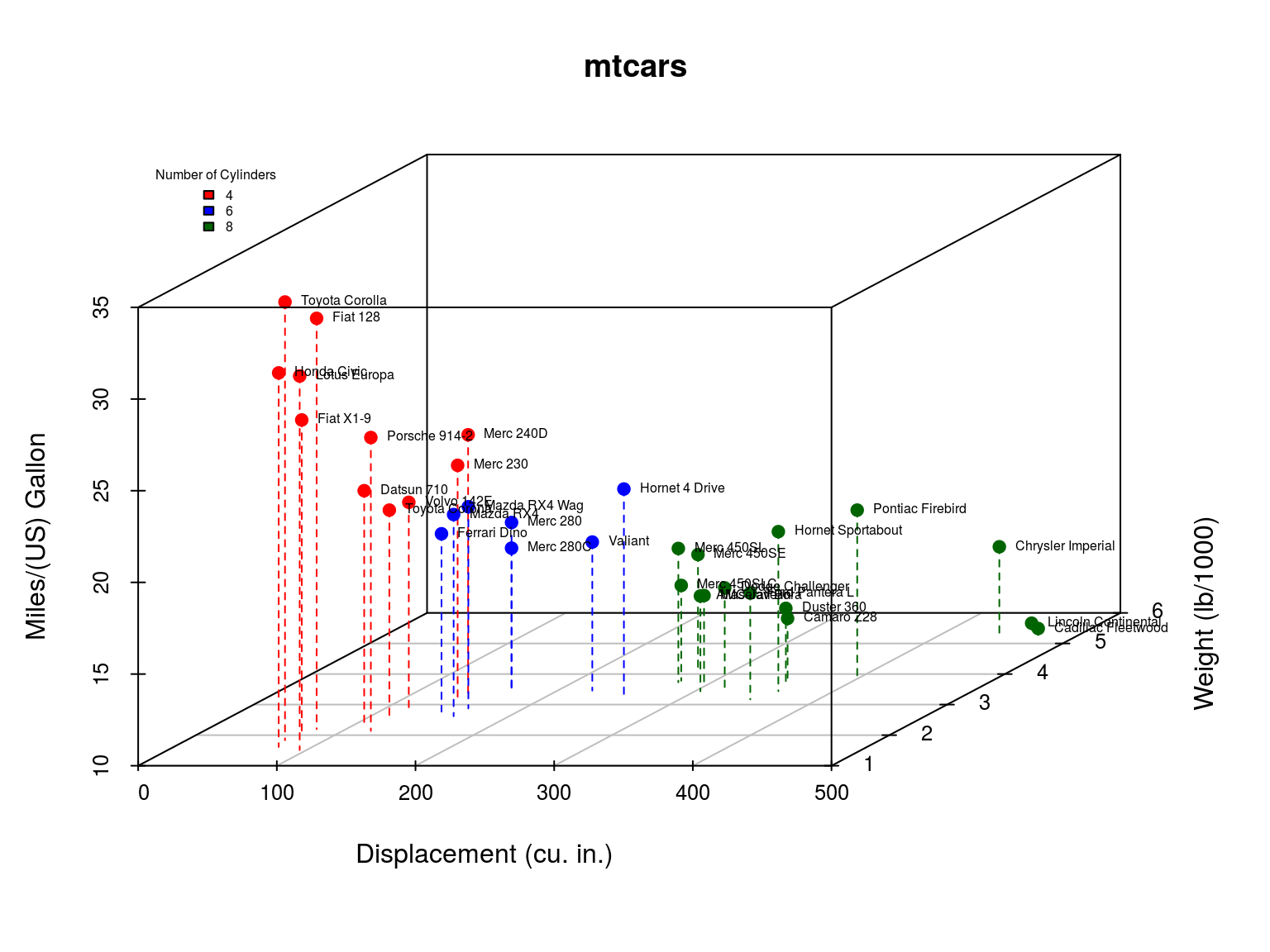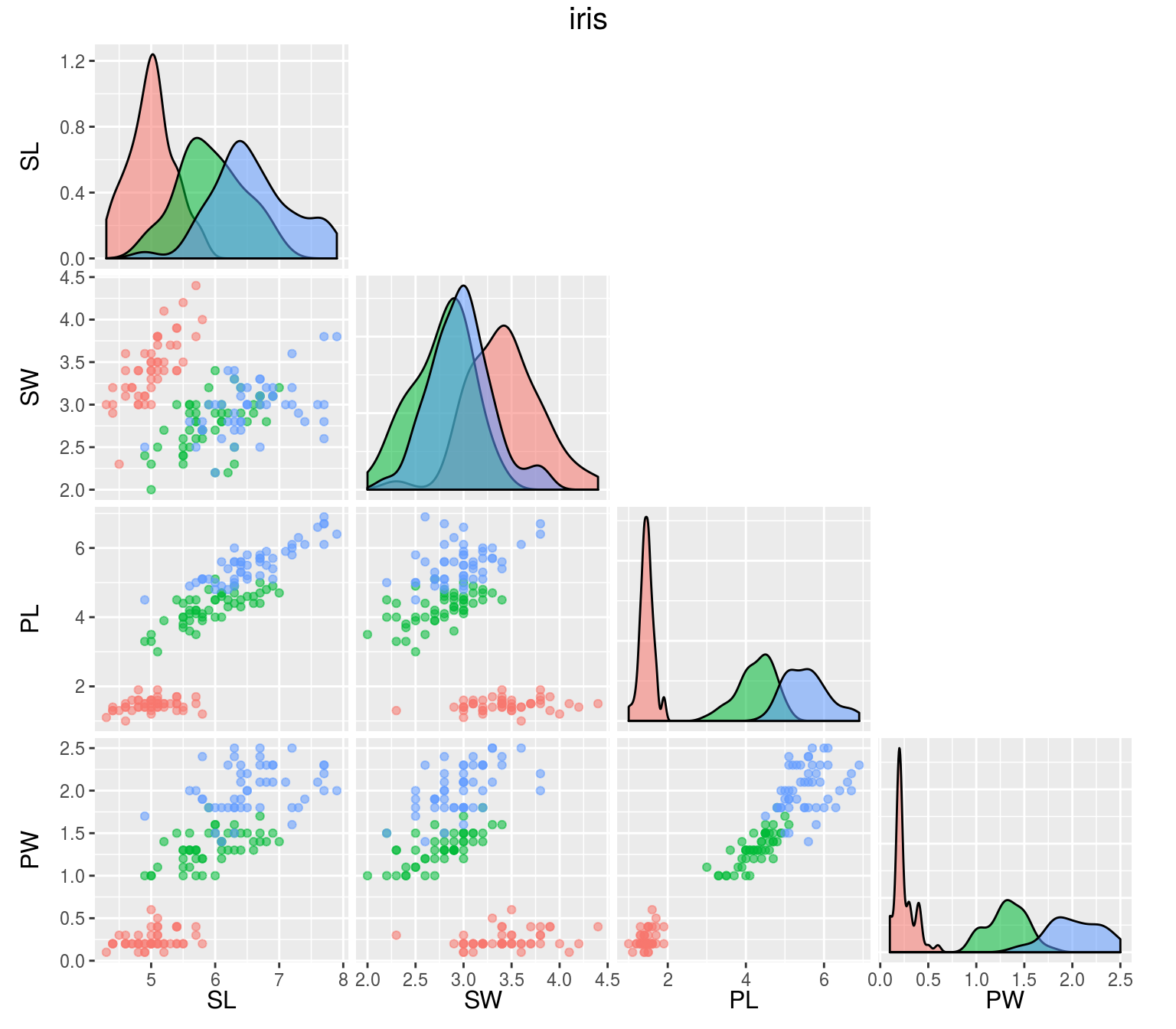## Dataset

mtcars: Fuel consumption and 10 aspects of automobile design and performance for 32 automobiles. A data frame with 32 observations on 11 variables:

• mpg: Miles/(US) gallon
• cyl: Number of cylinders
• disp: Displacement (cu.in.)
• hp: Gross horsepower
• drat: Rear axle ratio
• wt: Weight (1000 lbs)
• qsec: 1/4 mile time
• vs: V/S
• am: Transmission (0 = automatic, 1 = manual)
• gear: Number of forward gears
• carb: Number of carburetors
head(mtcars)
##                    mpg cyl disp  hp drat    wt  qsec vs am gear carb
## Mazda RX4         21.0   6  160 110 3.90 2.620 16.46  0  1    4    4
## Mazda RX4 Wag     21.0   6  160 110 3.90 2.875 17.02  0  1    4    4
## Datsun 710        22.8   4  108  93 3.85 2.320 18.61  1  1    4    1
## Hornet 4 Drive    21.4   6  258 110 3.08 3.215 19.44  1  0    3    1
## Hornet Sportabout 18.7   8  360 175 3.15 3.440 17.02  0  0    3    2
## Valiant           18.1   6  225 105 2.76 3.460 20.22  1  0    3    1iris: Gives the measurements in centimeters of the variables sepal length and width and petal length and width, respectively, for 50 flowers from each of 3 species of iris. A data frame with 150 cases (rows) and 5 variables (columns) named:

• Sepal.Length
• Sepal.Width
• Petal.Length
• Petal.Width
• Species
head(iris)
##   Sepal.Length Sepal.Width Petal.Length Petal.Width Species
## 1          5.1         3.5          1.4         0.2  setosa
## 2          4.9         3.0          1.4         0.2  setosa
## 3          4.7         3.2          1.3         0.2  setosa
## 4          4.6         3.1          1.5         0.2  setosa
## 5          5.0         3.6          1.4         0.2  setosa
## 6          5.4         3.9          1.7         0.4  setosa## OneR

Generates a one-level decision tree expressed in the form of a set of rules that all test one particular attribute. 1R chooses the attribute that produces rules with the smallest error rate.

mod.oner <- OneR(Species ~ ., data = iris)
print(mod.oner)
## Petal.Width:
##  < 0.8   -> setosa
##  < 1.75  -> versicolor
##  >= 1.75 -> virginica
## (144/150 instances correct)

## M5

Constructed by first using a decision tree induction algorithm minimizing intrasubset variation in the class values down each branch.

Splitting criterion: Maximize standard deviation reduction, $SDR = sd(T) − \sum_i{\frac{|T_i|}{|T|} * sd(T_i)},$ where $$T_1, T_2, ...$$ are the sets that result from splitting the node according to the chosen attribute, and $$sd(T)$$ is the standard deviation of the class values.

A linear model is built for each interior node of the tree and using a greedy search removes variables that contribute little. M5 then applies pruning by subtree replacement. And finally the prediction accuracy is improved by a smoothing process, $PV(S) = \frac{n_i \times PV(S_i) + k \times M(S)}{n_i + k},$ where $$PV(S_i)$$ is the predicted value at branch $$S_i$$ of subtree S,
$$M(S)$$ is the value given by the model at $$S$$,
$$n_i$$ is the number of training cases at $$S_i$$, and
$$k$$ is a smoothing constant.

mod.m5 <- M5Rules(mpg ~ ., data = mtcars)
print(mod.m5)
## M5 pruned model rules
## (using smoothed linear models) :
## Number of Rules : 2
##
## Rule: 1
## IF
##  cyl > 5
## THEN
##
## mpg =
##  -0.5389 * cyl
##  + 0.0048 * disp
##  - 0.0206 * hp
##  - 3.0997 * wt
##  + 34.4212 [21/26.733%]
##
## Rule: 2
##
## mpg =
##  -0.1351 * disp
##  + 40.872 [11/59.295%]

## RIPPER

RIPPER is a variant of the original IREP (incremental reduced error pruning, integration of reduced error pruning with a separate-and-conquer rule learning) algorithm with three modifications:

• Alternative metric for guiding its pruning phase: Deletes of any final sequence of conditions from the rule to maximize the function $v^*(Rule, PrunePos, PruneNeg) \equiv \frac{p - n}{p + n},$ where $$P$$ (respectively $$N$$) is the total number of examples in $$PrunePos$$ ($$PruneNeg$$) and $$p$$ ($$n$$) is the number of examples in $$PrunePos$$ ($$PruneNeg$$) covered by $$Rule$$.

• A new Stopping condition: After each rule is added, the total description length of the ruleset and the examples is computed, and stops adding rules when this description is more than d bits larger than the smallest description length obtained so far.

• Optimization of initial rules learned by IREP: Considered in the order they were constructed, for each rule $$R_i$$ two alternatives are constructed: a replacement $$R_i'$$ (exclude $$R_i$$ and minimize error of ruleset), and a revised $$R_i$$ (greedily adding conditions to $$R_i$$). A MDL heuristic is used to decide whether the final theory should include the replacement, revised, or original rule.

mod.rip <- JRip(Species ~ ., data = iris)
print(mod.rip)
## JRIP rules:
## ===========
##
## (Petal.Length <= 1.9) => Species=setosa (50.0/0.0)
## (Petal.Width <= 1.7) and (Petal.Length <= 4.9) => Species=versicolor (48.0/1.0)
##  => Species=virginica (52.0/3.0)
##
## Number of Rules : 3

## Remarks

Once the rules are created a domain expert can review and filter these rules to create a feasible subset. When working with large datasets, rules can be visualized for convenience of interpreting the model.